### Home > PC > Chapter 8 > Lesson 8.1.3 > Problem8-42

8-42.
1. Let g(x) =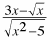. Your task is to find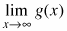. Outlined below are two different ways to find this limit with your calculator. You should try both of them. After you know how to do both, you can choose the method you prefer. For either approach, enter g(x) into [Y=] on your calculator. Homework Help ✎

1. Set the window on your calculator for 0 ≤ x ≤ 10,000,000, then graph.

2. You can also use the [TABLE] function. Set the [TBLSET] on your calculator to TblStart = 1,000,000 with ΔTbl = 1,000,000. Then read the table values.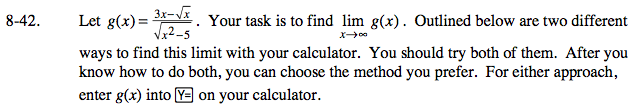By inspection using the Dominant Term Idea, the limit is 3.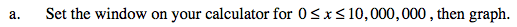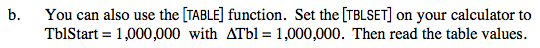Complete the table in the eTool below to help solve this problem.
Click the link at right for the full version of the eTool: PCT 8-42 HW eTool